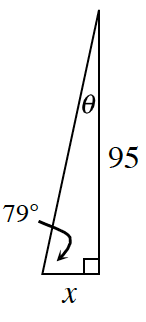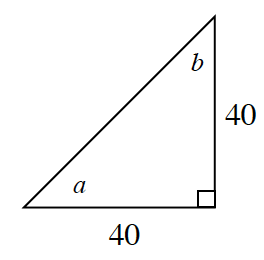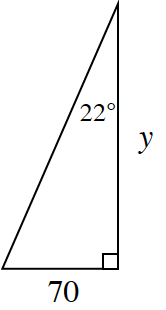### Home > INT2 > Chapter 3 > Lesson 3.2.2 > Problem3-83

3-83.

Use your Trig Table Graphic Organizer from problem 3-82 to help you determine the value of each variable below.

1.By the Triangle Angle Sum Theorem, $79^\circ + θ + 90^\circ = 180^\circ$

$θ = 11^\circ$

$\frac{95}{x}\approx \frac{5}{1}$

$x\approx19$

1.Notice that the legs of the right triangle are the same lengths.

1.Look at the hints in part (a).